x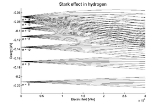Stark effectEncyclopedia"Stark shift" is not to be confused with Stokes shift
Stokes shift
Stokes shift is the difference between positions of the band maxima of the absorption and emission spectra of the same electronic transition. It is named after Irish physicist George G. Stokes. When a system absorbs a photon, it gains energy and enters an excited state...

.

The Stark effect is the shifting and splitting of spectral line
Spectral line
A spectral line is a dark or bright line in an otherwise uniform and continuous spectrum, resulting from a deficiency or excess of photons in a narrow frequency range, compared with the nearby frequencies.- Types of line spectra :...

s of atoms and molecules due to presence of an external static electric field
Electric field
In physics, an electric field surrounds electrically charged particles and time-varying magnetic fields. The electric field depicts the force exerted on other electrically charged objects by the electrically charged particle the field is surrounding...

. The amount of splitting and or shifting is called the Stark splitting or Stark shift. In general one distinguishes first- and second-order Stark effects. The first-order effect is linear in the applied electric field, while the second-order effect is quadratic in the field.

The Stark effect is responsible for the pressure broadening (Stark broadening) of spectral lines by charged particles. When the split/shifted lines appear in absorption, the effect is called the inverse Stark effect.

The Stark effect is the electric analogue of the Zeeman effect
Zeeman effect
The Zeeman effect is the splitting of a spectral line into several components in the presence of a static magnetic field. It is analogous to the Stark effect, the splitting of a spectral line into several components in the presence of an electric field...

where a spectral line is split into several components due to the presence of a magnetic field
Magnetic field
A magnetic field is a mathematical description of the magnetic influence of electric currents and magnetic materials. The magnetic field at any given point is specified by both a direction and a magnitude ; as such it is a vector field.Technically, a magnetic field is a pseudo vector;...

.

The Stark effect can be explained with fully quantum mechanical approaches, but it has also been a fertile testing ground for semiclassical methods.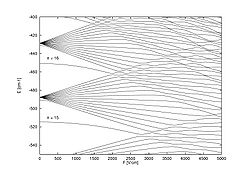## History

The effect is named after Johannes Stark
Johannes Stark
Johannes Stark was a German physicist, and Physics Nobel Prize laureate who was closely involved with the Deutsche Physik movement under the Nazi regime.-Early years:...

, who discovered it in 1913. It was independently discovered in the same year by the Italian physicist Antonino Lo Surdo
Antonino Lo Surdo
Antonino Lo Surdo was a famous Italian physicist. He was appointed as professor of physics at the Istituto di Fisica in Rome in 1919; upon the death of Orso Mario Corbino in 1937, he became the director...

, and in Italy it is thus sometimes called the Stark-Lo Surdo effect. The discovery of this effect contributed importantly to the development of quantum theory. Ironically, soon after their discoveries, both Stark and Lo Surdo rejected developments in modern physics and allied themselves with the political and racial programs of Hitler and Mussolini.

Inspired by the magnetic Zeeman effect
Zeeman effect
The Zeeman effect is the splitting of a spectral line into several components in the presence of a static magnetic field. It is analogous to the Stark effect, the splitting of a spectral line into several components in the presence of an electric field...

, and especially by Lorentz's explanation of it, Woldemar Voigt
Woldemar Voigt
Woldemar Voigt was a German physicist, who taught at the Georg August University of Göttingen. Voigt eventually went on to head the Mathematical Physics Department at Göttingen and was succeeded in 1914 by Peter Debye, who took charge of the theoretical department of the Physical Institute...

performed classical mechanical calculations of quasi-elastically bound electrons in an electric field. By using experimental indices of refraction he gave an estimate of the Stark splittings. This estimate was a few orders of magnitude too low. Not deterred by this prediction, Stark undertook measurements on excited states of the hydrogen atom and succeeded in observing splittings.

By the use of the Bohr-Sommerfeld ("old") quantum theory Paul Epstein
Paul Sophus Epstein
Paul Sophus Epstein was a Russian-American mathematical physicist...

and Karl Schwarzschild
Karl Schwarzschild
Karl Schwarzschild was a German physicist. He is also the father of astrophysicist Martin Schwarzschild.He is best known for providing the first exact solution to the Einstein field equations of general relativity, for the limited case of a single spherical non-rotating mass, which he accomplished...

were independently able to derive equations for the linear and quadratic Stark effect in hydrogen
Hydrogen
Hydrogen is the chemical element with atomic number 1. It is represented by the symbol H. With an average atomic weight of , hydrogen is the lightest and most abundant chemical element, constituting roughly 75% of the Universe's chemical elemental mass. Stars in the main sequence are mainly...

. Four years later, Hendrik Kramers
Hendrik Anthony Kramers
Hendrik Anthony "Hans" Kramers was a Dutch physicist.-Background and education:...

derived formulas for intensities of spectral transitions. Kramers also included the effect of fine structure
Fine structure
In atomic physics, the fine structure describes the splitting of the spectral lines of atoms due to first order relativistic corrections.The gross structure of line spectra is the line spectra predicted by non-relativistic electrons with no spin. For a hydrogenic atom, the gross structure energy...

, which includes corrections for relativistic kinetic energy and coupling between electron spin and orbit. The first quantum mechanical treatment (in the framework of Heisenberg's matrix mechanics
Matrix mechanics
Matrix mechanics is a formulation of quantum mechanics created by Werner Heisenberg, Max Born, and Pascual Jordan in 1925.Matrix mechanics was the first conceptually autonomous and logically consistent formulation of quantum mechanics. It extended the Bohr Model by describing how the quantum jumps...

) was by Wolfgang Pauli. Erwin Schrödinger discussed at length the Stark effect in his third paper on quantum theory (in which he introduced his perturbation theory), once in the manner of the 1916 work of Epstein (but generalized from the old to the new quantum theory) and once by his (first-order) perturbation approach.
Finally, Epstein reconsidered the linear and quadratic Stark effect from the point of view of the new quantum theory. He derived equations for the line intensities which were a decided improvement over Kramers' results obtained by the old quantum theory.

While first-order perturbation effects for the Stark effect in hydrogen are in agreement for the Bohr-Sommerfeld model and the quantum-mechanical
Quantum mechanics
Quantum mechanics, also known as quantum physics or quantum theory, is a branch of physics providing a mathematical description of much of the dual particle-like and wave-like behavior and interactions of energy and matter. It departs from classical mechanics primarily at the atomic and subatomic...

theory of the atom, higher order effects are not. Measurements of the Stark effect under high field strengths confirmed the correctness of the quantum theory over the Bohr model.

### Classical electrostatics

The Stark effect originates from the interaction between a charge distribution (atom or molecule) and an external electric field
Electric field
In physics, an electric field surrounds electrically charged particles and time-varying magnetic fields. The electric field depicts the force exerted on other electrically charged objects by the electrically charged particle the field is surrounding...

. Before turning to quantum mechanics we describe the interaction
classically and consider a continuous charge distribution ρ(r).
If this charge distribution is non-polarizable its interaction energy with an external electrostatic potential V(r) is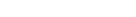.
If the electric field is of macroscopic origin and the charge distribution is microscopic, it is reasonable to assume that the electric field is uniform over the charge distribution. That is, V is given by a two-term Taylor expansion,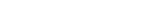, with the electric field: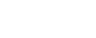,
where we took the origin 0 somewhere within ρ.
Setting V(0) as the zero energy, the interaction becomes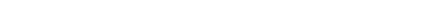.
Here we have introduced the dipole moment
Dipole
In physics, there are several kinds of dipoles:*An electric dipole is a separation of positive and negative charges. The simplest example of this is a pair of electric charges of equal magnitude but opposite sign, separated by some distance. A permanent electric dipole is called an electret.*A...

μ of ρ as an integral over the charge distribution. In case ρ consists of N point charges qj this definition becomes a sum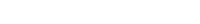.

### Perturbation theory

Turning now to quantum mechanics we see an atom or a molecule as a collection of point charges (electrons and nuclei), so that the second definition of the dipole applies. The interaction of atom or molecule with a uniform external field is described by the operator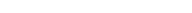This operator is used as a perturbation in first- and second-order perturbation theory
Perturbation theory
Perturbation theory comprises mathematical methods that are used to find an approximate solution to a problem which cannot be solved exactly, by starting from the exact solution of a related problem...

to account for the first- and second-order Stark effect.

#### First order

Let the unperturbed atom or molecule be in a g-fold degenerate state with orthonormal zeroth-order state functions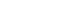. (Non-degeneracy is the special case g = 1). According to perturbation theory the first-order energies are the eigenvalues of the g x g matrix with general element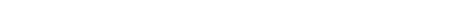If g = 1 (as is often the case for electronic states of molecules) the first-order energy becomes proportional to the expectation (average) value of the dipole operator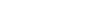,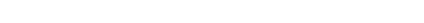Because a dipole moment is a polar vector, the diagonal elements of the perturbation matrix Vint vanish for systems with an inversion center
Parity (physics)
In physics, a parity transformation is the flip in the sign of one spatial coordinate. In three dimensions, it is also commonly described by the simultaneous flip in the sign of all three spatial coordinates:...

(such as atoms). Molecules with an inversion center in a non-degenerate electronic state do not have a (permanent) dipole and hence do not show a linear Stark effect.

In order to obtain a non-zero matrix Vint for systems with an inversion center it is necessary that some of the unperturbed functions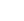have opposite parity (obtain plus and minus under inversion), because only functions of opposite parity give non-vanishing matrix elements. Degenerate zeroth-order states of opposite parity occur for excited hydrogen-like (one-electron) atoms. Such atoms have the principal quantum number n among their quantum numbers. The excited state of hydrogen-like atoms with principal quantum number n is n2-fold degenerate and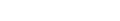where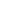is the azimuthal (angular momentum) quantum number. For instance, the excited n = 4 state contains the followingstates,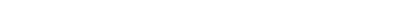The one-electron states with evenare even under parity, while those with oddare odd under parity. Hence hydrogen-like atoms with n>1 show first-order Stark effect.

The first-order Stark effect occurs in rotational transitions of symmetric top molecules (but not for linear and asymmetric molecules). In first approximation a molecule may be seen as a rigid rotor. A symmetric top rigid rotor
Rigid rotor
The rigid rotor is a mechanical model that is used to explain rotating systems.An arbitrary rigid rotor is a 3-dimensional rigid object, such as a top. To orient such an object in space three angles are required. A special rigid rotor is the linear rotor which isa 2-dimensional object, requiring...

has the unperturbed eigenstates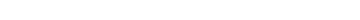with 2(2J+1)-fold degenerate energy for |K| > 0 and (2J+1)-fold degenerate energy for K=0.
Here DJMK is an element of the Wigner D-matrix
Wigner D-matrix
The Wigner D-matrix is a matrix in an irreducible representation of the groups SU and SO. The complex conjugate of the D-matrix is an eigenfunction of the Hamiltonian of spherical and symmetric rigid rotors. The matrix was introduced in 1927 by Eugene Wigner.- Definition Wigner D-matrix :Let j_x,...

. The first-order perturbation matrix on basis of the unperturbed rigid rotor function is non-zero and can be diagonalized. This gives shifts and splittings
in the rotational spectrum. Quantitative analysis of these Stark shift yields the permanent electric dipole moment
Electric dipole moment
In physics, the electric dipole moment is a measure of the separation of positive and negative electrical charges in a system of charges, that is, a measure of the charge system's overall polarity with SI units of Coulomb-meter...

of the symmetric top molecule.

#### Second order

As stated, the quadratic Stark effect is described by second-order perturbation theory.
The zeroth-order problems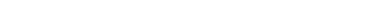are assumed to be solved. It is usual to assume that the zeroth-order state to be perturbed is non-degenerate. If we take the ground state as the non-degenerate state under consideration (for hydrogen-like atoms: n = 1), perturbation theory gives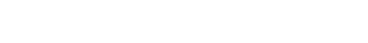with the components of the polarizability
Polarizability
Polarizability is the measure of the change in a molecule's electron distribution in response to an applied electric field, which can also be induced by electric interactions with solvents or ionic reagents. It is a property of matter...

tensor α defined by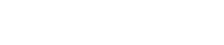The energy E(2) gives the quadratic Stark effect.

Because of their spherical symmetry the polarizability tensor of atoms is isotropic,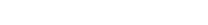which is the quadratic Stark shift for atoms. For many molecules this expression is not too bad an approximation, because molecular tensors are often reasonably isotropic.

#### Problems

The perturbative treatment of the Stark effect has some problems. In the presence of an electric field, states of atoms and molecules that were previously bound (square-integrable), become formally (non-square-integrable) resonance
Resonance
In physics, resonance is the tendency of a system to oscillate at a greater amplitude at some frequencies than at others. These are known as the system's resonant frequencies...

s of finite width.
These resonances may decay in finite time via field ionization. For low lying states and not too strong fields the decay times are so long, however, that for all practical purposes the system can be regarded as bound. For highly excited states and/or very strong fields ionization may have to be accounted for. (See also the article on the Rydberg atom
Rydberg atom
thumb|right|300px|Figure 1: Energy levels in atomic [[lithium]] showing the Rydberg series of the lowest 3 values of [[Angular momentum#Angular momentum in quantum mechanics|orbital angular momentum]] converging on the first ionization energy....

).

## Quantum-confined Stark effect

In a semiconductor heterostructure, where a small bandgap material is sandwiched between two layers of a larger bandgap material, the Stark effect can be dramatically enhanced by bound excitons. This is because the electron
Electron
The electron is a subatomic particle with a negative elementary electric charge. It has no known components or substructure; in other words, it is generally thought to be an elementary particle. An electron has a mass that is approximately 1/1836 that of the proton...

and hole
Electron hole
An electron hole is the conceptual and mathematical opposite of an electron, useful in the study of physics, chemistry, and electrical engineering. The concept describes the lack of an electron at a position where one could exist in an atom or atomic lattice...

which form the exciton are pulled in opposite directions by the applied electric field, but they remain confined in the smaller bandgap material, so the exciton is not merely pulled apart by the field. The quantum-confined Stark effect is widely used for semiconductor-based optical modulators, particularly for optical fiber
Optical fiber
An optical fiber is a flexible, transparent fiber made of a pure glass not much wider than a human hair. It functions as a waveguide, or "light pipe", to transmit light between the two ends of the fiber. The field of applied science and engineering concerned with the design and application of...

communications.

• Zeeman splitting
• Autler-Townes effect
Autler-Townes effect
In spectroscopy, the Autler-Townes effect is a type of the dynamical Stark effect, corresponding to the case when an oscillating electric field is tuned in resonance to the transition frequency of a given spectral line, and resulting in a change of the shape of the absorption/emission spectra of...

• Stark spectroscopy
Stark spectroscopy
Stark spectroscopy is a form of spectroscopy based on the Stark effect. In brief, this technique makes use of the Stark effect either to reveal information about the physiochemical or physical properties of a sample using a well-characterized electric field or to reveal information about an...

• Inglis-Teller Equation
Inglis-Teller equation
The Inglis–Teller equation represents an approximate relationship between an energy level and the electron number density. This equation is related to the Stark effect in which spectral lines are split explicitly due to the presence of an electric field. The equation was derived by David R...